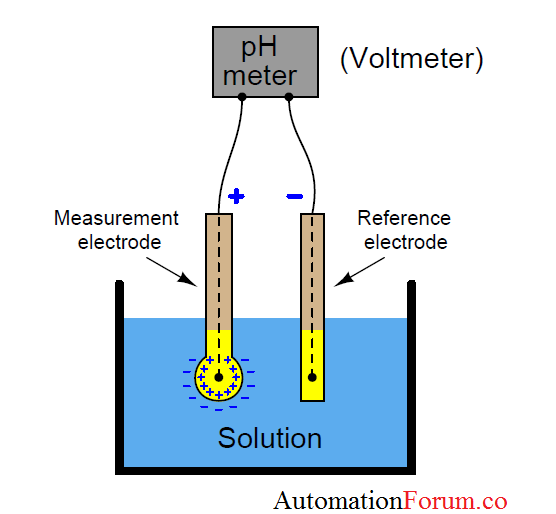# pH measurement### What is pH?

pH is a unit of measurement that describes the degree of acidity or alkali content of a solution. The pH unit is measured in terms of 0 to 14.

The pH is formed from quantitative information expressed by the level of acidity or base associated with the activity of hydrogen ions.

If the concentration [H +] is greater than [OH-], then the material is acidic, i.e. the pH value is less than 7. If the concentration of [OH-] is greater than [H +], then the material is alkaline, with a pH value greater than 7.

### pH meter:

The pH measurement system has three parts, namely the pH measurement electrode, the reference electrode, and the high impedance measuring device

PH meter (pH electrode) is an electronic instrument used for measuring pH (acidity) of a solution (although it can also be used for semi-solid pH measurement ).

#### pH measurement principle

The measurement of a pH is based on the electro chemical potential that occurs between solutions found in glass electrodes (glass membranes) which have been known to dissolve which exist outside unknown glass electrodes

This is because a thin layer of glass bubbles will interact with hydrogen which is relatively small in size and active, the electrode will measure the electrochemical potential of hydrogen ions or term with the potential of hydrogen.

### pH meter working:

The pH meter will measure the electrical potential (in a clockwise flow image) between mercury chloride (HgCl) on the electrodes of comparison and potassium chloride (KCl) which is dissolved in the glass electrode and the potential between the solution.

The glass electrode consists of a sturdy glass tube which is connected with a thin glass bubble. Inside there is a KCl solution as a pH buffer. The tip of the silver electrode is silver chloride (AgCl2) connected to the solution

To minimize the electric effect that is not desired, the tool is protected by a layer of protective paper which is usually found in glass electrodes.

In most modern pH meters it is equipped with temperature thermistor which is a tool to correct temperature influences. Between the comparative electrodes and the gel electrodes are arranged in one unit.

Scroll to Top